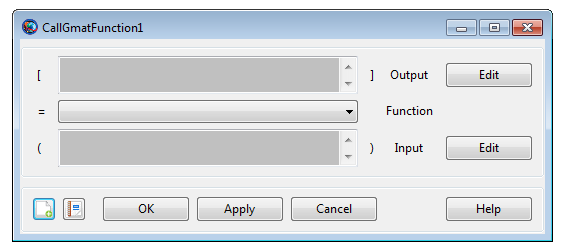# CallGmatFunction

## CallGmatFunction

CallGmatFunction — Call a GMAT function

## Script Syntax

GmatFunction()
GmatFunction(input_argument[, input_argument]...)
[output_argument[, output_argument]...] = GmatFunction
[output_argument[, output_argument]...] = ...
GmatFunction(input_argument[, input_argument]...)

## Description

GMAT provides a special command that allows you to call a GMAT function which is written via GMAT's GmatFunction resource. In the GUI, the GMAT function is called through the CallGmatFunction command.

In the syntax description, GmatFunction is a GmatFunction resource that must be declared during initialization. Arguments can be passed into the function as inputs and returned from the function as outputs. See Remarks for details. Furthermore, data that is passed into the function as input or received from the function as output can also be declared as global by using GMAT's Global command. See the Global reference for more details.

See Also: GMATFunction, Global

## GUIThe CallGmatFunction GUI provides two input boxes for input and output arguments and a list to select a GMAt function to call.

The Output box lists all configured output argument parameters. These must be selected by clicking Edit, which displays a ParameterSelectioDialog window. See the Calculation Parameters reference for details on how to select a parameter.

The Input box is identical in behavior to Output, but lists all configured input arguments to the function. Arguments must be selected by clicking Edit. The Function list displays all functions that have been declared as GmatFunction resources in the Resources tree. Select a function from the list to call it.

When the changes are accepted, GMAT does not perform any validation of input or output arguments. This validation is performed when the mission is actually run.

## Remarks

GMAT objects can be passed into the GMAT function as input and can also be returned from the function as output. If a given GMAT object is not declared as global in both the main script and inside the GMAT function, then all objects that are passed into or received as output from the function are considered to be local to that function and the main script.

Below is a list of allowed arguments that can be passed as input to the function and received as output from the function. Also see GmatFunction resource's Remarks and Examples sections for more details and distinct examples that show how to pass objects as inputs to the function, perform an operation inside the function, then receive objects as outputs from the function. Note, a GMAT function file must contain one and only one function definition.

The input arguments (input_argument values in the syntax description) can be any of the following types:

• Any resource objects (e.g. Spacecraft, Propagator, DC, Optimizers, Impulsive or FiniteBurns)

• resource parameter of real number type (e.g. Spacecraft.X)

• resource parameter of string type (e.g. Spacecraft.UTCGregorian)

• Array, String, or Variable resource

The output arguments can be any of the following types:

• Resource object like Spacecraft

• resource parameter of real number type (e.g. Spacecraft.X)

• resource parameter of string type (e.g. Spacecraft.UTCGregorian)

• Array, String, or Variable resource

## Examples

Call two different functions. One function performs a simple cross product and the second function performs a dot product.

          Create ReportFile rf
rf.WriteHeaders = false

Create GmatFunction cross_product
cross_product.FunctionPath = ...
'C:\Users\rqureshi\Desktop\cross_product.gmf'

Create GmatFunction dot_product
dot_product.FunctionPath = ...
'C:\Users\rqureshi\Desktop\dot_product.gmf'

Create Array v1[3,1] v2[3,1] v3[3,1] ...
v4[3,1] v5[3,1]

Create Variable v6
Create String tempstring

BeginMissionSequence

v1(1,1) = 1
v1(2,1) = 2
v1(3,1) = 3
v2(1,1) = 4
v2(2,1) = 5
v2(3,1) = 6
v4(1,1) = 1
v4(2,1) = 2
v4(3,1) = 3
v5(1,1) = 4
v5(2,1) = -5
v5(3,1) = 6

% Call function. Pass local arrays as input:
% Receive local array as output
[v3] = cross_product(v1, v2)

Report rf v3

% Call function. Pass local arrays as input:
% Receive local variable as output
GMAT [v6] = dot_product(v4, v5)

tempstring = '---------'
Report rf tempstring
Report rf v6

%%%%%% cross_product Function begins below:

function [cross] = cross_product(vec1,vec2)

Create Array cross[3,1]

BeginMissionSequence

cross(1,1) = vec1(2,1)*vec2(3,1) - vec1(3,1)*vec2(2,1)
cross(2,1) = -(vec1(1,1)*vec2(3,1) - vec1(3,1)*vec2(1,1))
cross(3,1) = vec1(1,1)*vec2(2,1) - vec1(2,1)*vec2(1,1)

%%%%%% dot_product Function begins below:

function [c] = dot_product(a1,b1)

Create Variable c

BeginMissionSequence

c = a1(1,1)*b1(1,1) + a1(2,1)*b1(2,1) + a1(3,1)*b1(3,1)


Call GMAT function and pass local spacecraft as input, perform simple operation inside the function, then send out updated, local spacecraft to the main script. Finally report spacecraft old and updated position vector to the local report file subscriber:

          Create Spacecraft aSat
aSat.DateFormat = UTCGregorian;
aSat.Epoch = '01 Jan 2000 11:59:28.000'
aSat.CoordinateSystem = EarthMJ2000Eq
aSat.DisplayStateType = Cartesian
aSat.X = 7100
aSat.Y = 0
aSat.Z = 1300

Create ReportFile rf
rf.WriteHeaders = false

Create GmatFunction Spacecraft_In_Out
Spacecraft_In_Out.FunctionPath = ...
'C:\Users\rqureshi\Desktop\Spacecraft_In_Out.gmf'

BeginMissionSequence

% Report initial S/C Position to local 'rf':
Report rf aSat.X aSat.Y aSat.Z

% Call function. Pass local S/C as input:
% Receive updated local S/C:
[aSat] = Spacecraft_In_Out(aSat)

% Report updated S/C Position to local 'rf':
Report rf aSat.X aSat.Y aSat.Z

%%%%%%%%%% Function begins below:

function [aSat] = Spacecraft_In_Out(aSat)

% Create local S/C:
Create Spacecraft aSat

BeginMissionSequence

% Update the S/C Position vector:
% Send updated S/C back to main script:
aSat.X = aSat.X + 1000
aSat.Y = aSat.Y + 2000
aSat.Z = aSat.Z + 3000